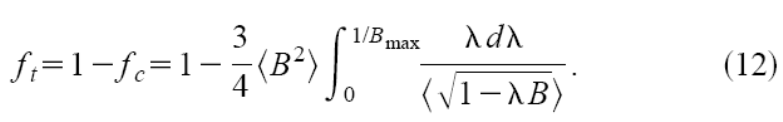Accurate neoclassical resistivity, bootstrap current and other transport coefficients

(Fortran 90 subroutines and matlab functions)

The Fokker-Planck codes CQL3D  and CQLP  have been used to calculate the neoclassical transport coefficients valid for arbitrary tokamak geometry and collisionality. These results provide better and more complete coefficients than the previously used Hirshman, Hinton, Chang, Taguchi, etc models.

We have fitted the exact Fokker-Planck results to a very good accuracy while maintaining relatively simple formulas.

This was possible because we found that the equilibrium effects can be very well encapsulated through a trapped fraction ft (which definition depends on the related transport coefficients [3: C. Angioni et al + errata]).

On the other hand, the collisionality effects can be well encapsulated through modifications of the trapped fraction, leading to effective trapped fractions.

The neoclassical conductivity sigmaneo and bootstrap current <jBS B> are given in the paper  O. Sauter et al and its errata (+0.315 instead of -0.315 in alpha coefficient)

The other neoclassical transport coefficients, like chi_i for example, are given in  C. Angioni et al (+ Errata, refs corrected), also valid for arbitrary geometry, aspect ratio and collisionality.

The input data are the profiles and the trapped fraction. The trapped fraction for conductivity and bootstrap, ft, can be obtained with either of:

1)     from the full formula as a double integral (as done in CHEASE), Eq. (12) of :2)     From the approximate formula by Lin-Liu using simple flux surface average of B's, thus only single integrals: Ref. 

3)     For most tokamak cases the following formula works relatively well (Eq. (4) of Ref ):Modules have been developed and are included in the CHEASE code for example. They are available here below:

F90 and matlab routines/modules for neoclassical resistivity and bootstrap current: NEOCLASSICAL.tar

·       How to use the formulas with experimental data and the questions of Z, Zeff

The original BS paper [4a] was not precise enough about the pressure, Ti, Z and Zeff. Additional information is given in the Errata [4b]. In particular:

o       Z = Zeff in all equations except Eq. 18(c) and Eq. 18(e) where Z=Zion (thus Z=1 for D) should be used

o       Total pressure should be used for p and pe/p should be used where stated and not some approximations with Te and Ti for example

o       The formula for ln L should be used as defined in the paper (Eqs 18(d,e))

The above are due to the fact that the fits to the exact code results have been performed using these definitions. The above statements are particularly true if a code benchmark is being performed.

The code results for various Zeff have been obtained using a single ion species with Zeff and therefore also assuming TI=Ti. This is a limitation which needs to be tested against experimental results. There are several comparisons with reconstructed profiles. The latest detailed comparison has been performed on AUG . In that case they assumed a single impurity and Zeff to define a total ni=nimain + niimp and to define total pressure. When ion temperature and/or impurity temperature were not available, Ti=Te, TI=Ti were used. It is shown in Ref.  that the edge bootstrap current is well predicted.

In smaller inverse aspect ration in particular, a correction is necessary near the edge as proposed recently in Ref.  and NSTX. This correction should be added in all cases, in particular in H-mode for the pedestal. A useful discussion of Z, Zeff is also given in Sec. V.

As a general statement and in the absence of specific more precise calculations, additional effects should be added in total pressure and equivalent Zeff, inspired by MHD effects. For example, fast particles contribution should be included in total pressure and therefore total pi be used as well.

References: